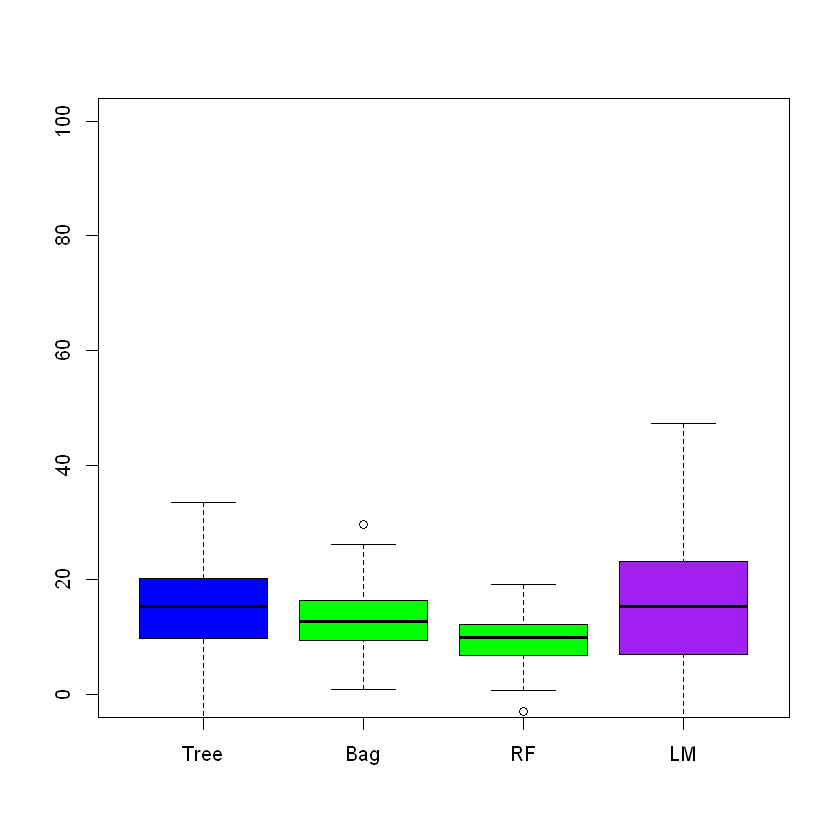# Bootstrap Example¶

In :
x<-c(1:100)
B<-100
p_boot <- numeric(B)
for(b in 1:B) {
## Take bootstrap sample and record the win proportion.
p_boot[b] <- mean(sample(x, replace = TRUE))
}

In :
p_boot

1. 52.21
2. 48.87
3. 52.57
4. 54.18
5. 47.46
6. 52.89
7. 52.28
8. 45.08
9. 50.94
10. 41.49
11. 52.73
12. 49.42
13. 51.37
14. 56.16
15. 47.75
16. 50.12
17. 51.24
18. 54.35
19. 47.33
20. 50.23
21. 47.66
22. 48.63
23. 50.27
24. 53.45
25. 51.17
26. 49.63
27. 53.66
28. 48.48
29. 46.57
30. 51.14
31. 52.36
32. 49.77
33. 49.99
34. 47.74
35. 50.33
36. 46.82
37. 53.29
38. 50.2
39. 50.8
40. 54.8
41. 50.4
42. 51.57
43. 50.9
44. 49.88
45. 56.91
46. 52.41
47. 49.39
48. 52.06
49. 55.62
50. 49.25
51. 47.03
52. 51.64
53. 53.16
54. 47.93
55. 53.62
56. 44.43
57. 54.43
58. 53.86
59. 45.42
60. 46.91
61. 51.54
62. 49.22
63. 56.04
64. 54.21
65. 49.46
66. 51.61
67. 53.81
68. 45.99
69. 49.98
70. 46.08
71. 50.63
72. 47.02
73. 48.36
74. 53.48
75. 46.2
76. 48.53
77. 50.23
78. 50.96
79. 48.2
80. 47.32
81. 55.18
82. 51.23
83. 51.49
84. 57.82
85. 54.09
86. 51.41
87. 49.24
88. 52.42
89. 51.15
90. 53.05
91. 51.94
92. 50.64
93. 50.05
94. 55.52
95. 51.58
96. 49.85
97. 49.04
98. 45.17
99. 48.45
100. 54.03
In :
mean(p_boot)

50.6447
In :
mean(x)

50.5

# Illustrative Example¶

In :
### Generate Data
x<-3*runif(100,1,5)
y<-sin(x)
noise_sample <- sample(1:length(y),15)
y[noise_sample]= 2*(0.5 - runif(15,-1,0.3))
df<-cbind(x,y)
df<-data.frame(df)
plot(y~x,col="blue")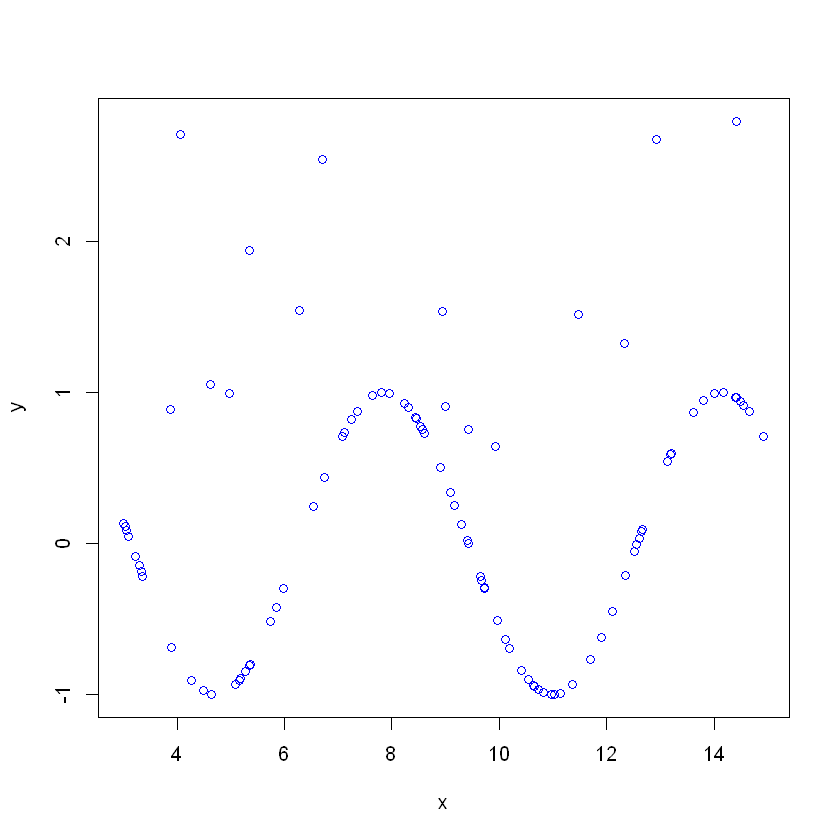In :
library(tree)
require(randomForest)
tree.df = tree(y~.,df)
prune.df =prune.tree(tree.df ,best =3)
rf.df =randomForest(y~.,df)
yhat.rf=predict (rf.df ,newdata =df)
yhat.tree=predict (tree.df ,newdata =df)
plot(tree.df)
text(tree.df,pretty =0)
tree.df

node), split, n, deviance, yval
* denotes terminal node

1) root 100 82.4300  0.24960
2) x < 12.7883 84 62.6800  0.09127
4) x < 9.53549 55 41.0500  0.33580
8) x < 6.13105 27 22.5700 -0.12870
16) x < 4.17296 11  8.1060  0.23960 *
17) x > 4.17296 16 11.9500 -0.38190 *
9) x > 6.13105 28  7.0340  0.78370
18) x < 9.03951 22  4.4500  0.92980 *
19) x > 9.03951 6  0.3925  0.24810 *
5) x > 9.53549 29 12.1100 -0.37250
10) x < 11.4208 18  3.2000 -0.65180
20) x < 9.9394 5  0.6552 -0.08283 *
21) x > 9.9394 13  0.3032 -0.87070 *
11) x > 11.4208 11  5.2070  0.08463 *
3) x > 12.7883 16  6.5850  1.08100 *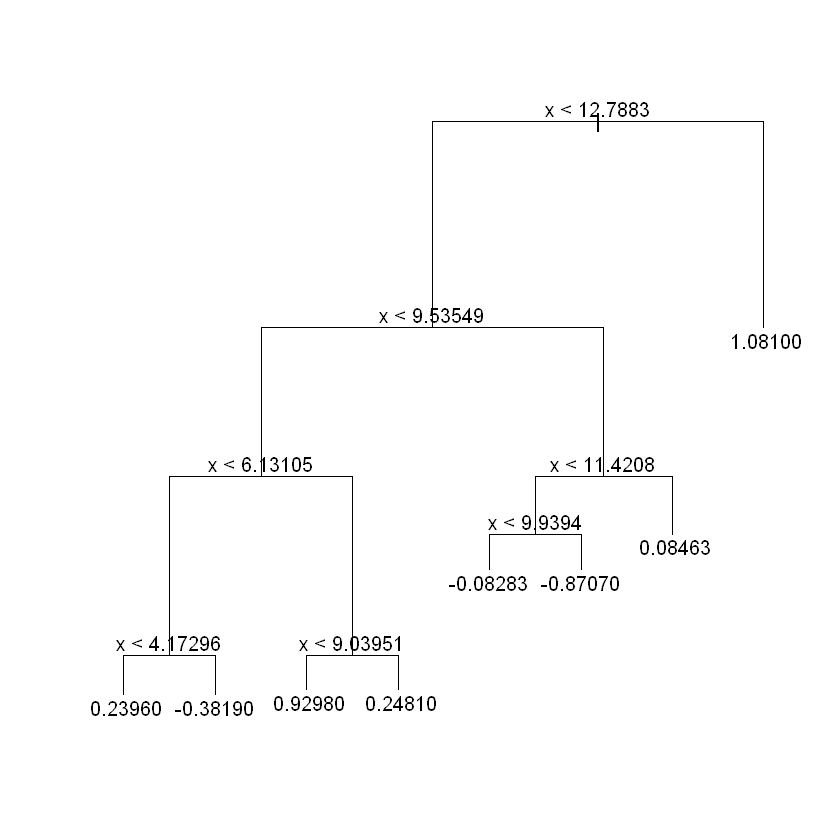In :
plot(y~x,col="blue",pch=18)
points(yhat.tree~x,col="red",pch=19)
#points(yhat.rf~x,col="green",pch=20)
#legend("bottomleft",legend=c("y","Tree-y","RF-Y"),
#      col=c("blue","red","green"),pch=18:19)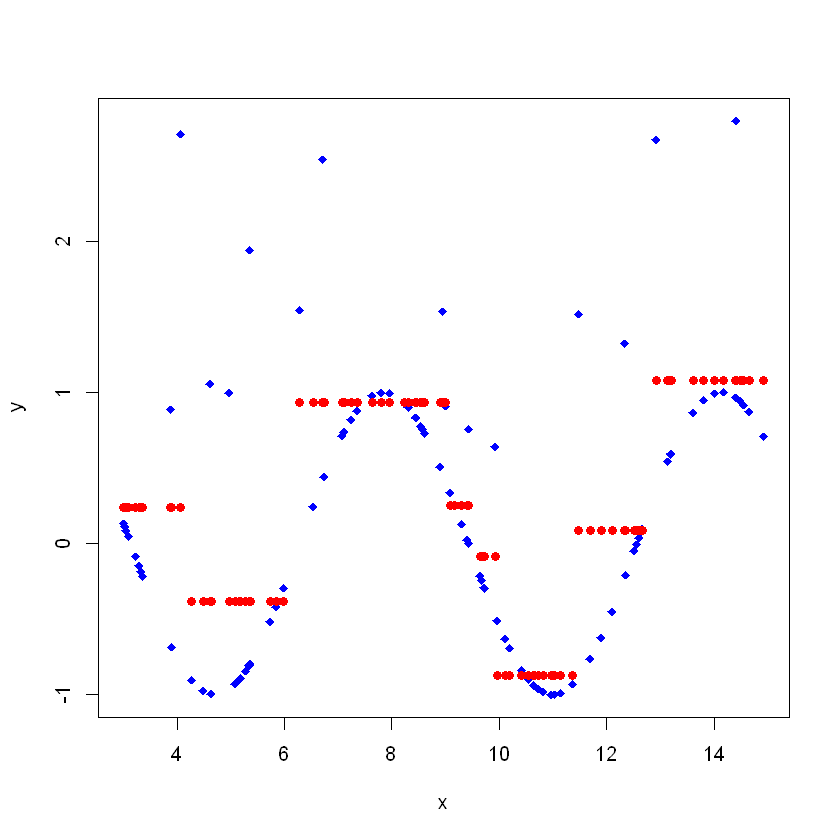In :
prune.df =prune.tree(tree.df ,best =3)
yhat.tree.p=predict (prune.df ,newdata =df)
plot(prune.df)
text(prune.df,pretty =0)
tree.df

node), split, n, deviance, yval
* denotes terminal node

1) root 100 82.4300  0.24960
2) x < 12.7883 84 62.6800  0.09127
4) x < 9.53549 55 41.0500  0.33580
8) x < 6.13105 27 22.5700 -0.12870
16) x < 4.17296 11  8.1060  0.23960 *
17) x > 4.17296 16 11.9500 -0.38190 *
9) x > 6.13105 28  7.0340  0.78370
18) x < 9.03951 22  4.4500  0.92980 *
19) x > 9.03951 6  0.3925  0.24810 *
5) x > 9.53549 29 12.1100 -0.37250
10) x < 11.4208 18  3.2000 -0.65180
20) x < 9.9394 5  0.6552 -0.08283 *
21) x > 9.9394 13  0.3032 -0.87070 *
11) x > 11.4208 11  5.2070  0.08463 *
3) x > 12.7883 16  6.5850  1.08100 *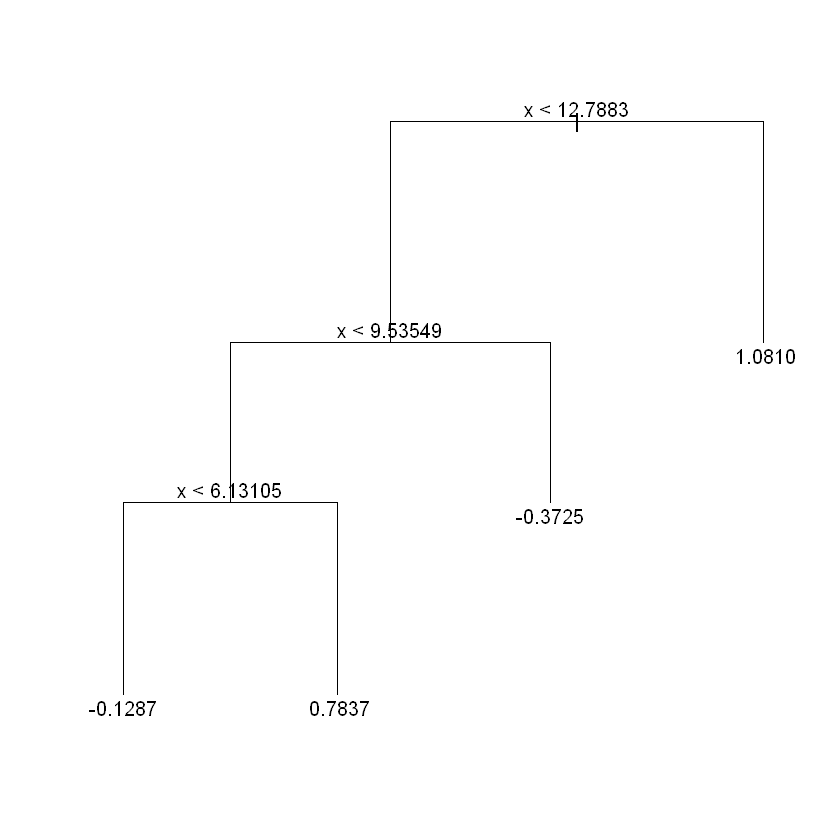In :
plot(y~x,col="blue",pch=18)
points(yhat.tree~x,col="red",pch=19)
points(yhat.tree.p~x,col="green",pch=20)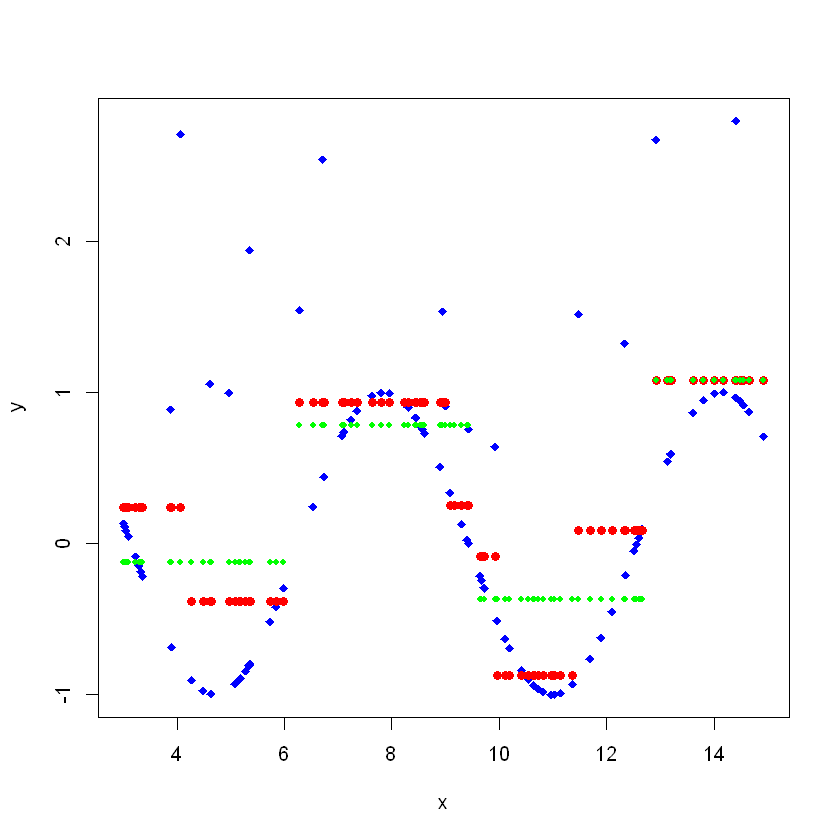# Data Analysis¶

In this section we are going to use tree based methods to predict the medium house price. We are going to use Boston house prices dataset. This data frame contains the following columns:

• crim -per capita crime rate by town.
• zn -proportion of residential land zoned for lots over 25,000 sq.ft.
• indus - proportion of non-retail business acres per town.
• chas - Charles River dummy variable (= 1 if tract bounds river; 0 otherwise).
• nox -nitrogen oxides concentration (parts per 10 million).
• rm- average number of rooms per dwelling.
• age - proportion of owner-occupied units built prior to 1940.
• dis - weighted mean of distances to five Boston employment centres.
• rad - index of accessibility to radial highways.
• tax - full-value property-tax rate per $\$10,000$. • ptratio - pupil-teacher ratio by town. • black -$1000(Bk−0.63)^2$where Bk is the proportion of blacks by town. • lstat - lower status of the population (percent). • medv - median value of owner-occupied homes in$1000s.

The tree library is used to construct classification and regression trees.

In :
#install.packages("tree")
library(tree)


Here we fit a regression tree to the Boston dataset.

In :
library(MASS)

0.0063218 2.31 0 0.538 6.575 65.2 4.0900 1 296 15.3 396.90 4.98 24.0
0.02731 0 7.07 0 0.469 6.421 78.9 4.9671 2 242 17.8 396.90 9.14 21.6
0.02729 0 7.07 0 0.469 7.185 61.1 4.9671 2 242 17.8 392.83 4.03 34.7
0.03237 0 2.18 0 0.458 6.998 45.8 6.0622 3 222 18.7 394.63 2.94 33.4
0.06905 0 2.18 0 0.458 7.147 54.2 6.0622 3 222 18.7 396.90 5.33 36.2
0.02985 0 2.18 0 0.458 6.430 58.7 6.0622 3 222 18.7 394.12 5.21 28.7

Check missing values

In :
sum(is.na(Boston))

0
In :
dim(Boston)

1. 506
2. 14
In :
summary(Boston)

      crim                zn             indus            chas
Min.   : 0.00632   Min.   :  0.00   Min.   : 0.46   Min.   :0.00000
1st Qu.: 0.08204   1st Qu.:  0.00   1st Qu.: 5.19   1st Qu.:0.00000
Median : 0.25651   Median :  0.00   Median : 9.69   Median :0.00000
Mean   : 3.61352   Mean   : 11.36   Mean   :11.14   Mean   :0.06917
3rd Qu.: 3.67708   3rd Qu.: 12.50   3rd Qu.:18.10   3rd Qu.:0.00000
Max.   :88.97620   Max.   :100.00   Max.   :27.74   Max.   :1.00000
nox               rm             age              dis
Min.   :0.3850   Min.   :3.561   Min.   :  2.90   Min.   : 1.130
1st Qu.:0.4490   1st Qu.:5.886   1st Qu.: 45.02   1st Qu.: 2.100
Median :0.5380   Median :6.208   Median : 77.50   Median : 3.207
Mean   :0.5547   Mean   :6.285   Mean   : 68.57   Mean   : 3.795
3rd Qu.:0.6240   3rd Qu.:6.623   3rd Qu.: 94.08   3rd Qu.: 5.188
Max.   :0.8710   Max.   :8.780   Max.   :100.00   Max.   :12.127
Min.   : 1.000   Min.   :187.0   Min.   :12.60   Min.   :  0.32
1st Qu.: 4.000   1st Qu.:279.0   1st Qu.:17.40   1st Qu.:375.38
Median : 5.000   Median :330.0   Median :19.05   Median :391.44
Mean   : 9.549   Mean   :408.2   Mean   :18.46   Mean   :356.67
3rd Qu.:24.000   3rd Qu.:666.0   3rd Qu.:20.20   3rd Qu.:396.23
Max.   :24.000   Max.   :711.0   Max.   :22.00   Max.   :396.90
lstat            medv
Min.   : 1.73   Min.   : 5.00
1st Qu.: 6.95   1st Qu.:17.02
Median :11.36   Median :21.20
Mean   :12.65   Mean   :22.53
3rd Qu.:16.95   3rd Qu.:25.00
Max.   :37.97   Max.   :50.00  
In :
Boston$chas  1. 0 2. 0 3. 0 4. 0 5. 0 6. 0 7. 0 8. 0 9. 0 10. 0 11. 0 12. 0 13. 0 14. 0 15. 0 16. 0 17. 0 18. 0 19. 0 20. 0 21. 0 22. 0 23. 0 24. 0 25. 0 26. 0 27. 0 28. 0 29. 0 30. 0 31. 0 32. 0 33. 0 34. 0 35. 0 36. 0 37. 0 38. 0 39. 0 40. 0 41. 0 42. 0 43. 0 44. 0 45. 0 46. 0 47. 0 48. 0 49. 0 50. 0 51. 0 52. 0 53. 0 54. 0 55. 0 56. 0 57. 0 58. 0 59. 0 60. 0 61. 0 62. 0 63. 0 64. 0 65. 0 66. 0 67. 0 68. 0 69. 0 70. 0 71. 0 72. 0 73. 0 74. 0 75. 0 76. 0 77. 0 78. 0 79. 0 80. 0 81. 0 82. 0 83. 0 84. 0 85. 0 86. 0 87. 0 88. 0 89. 0 90. 0 91. 0 92. 0 93. 0 94. 0 95. 0 96. 0 97. 0 98. 0 99. 0 100. 0 101. 0 102. 0 103. 0 104. 0 105. 0 106. 0 107. 0 108. 0 109. 0 110. 0 111. 0 112. 0 113. 0 114. 0 115. 0 116. 0 117. 0 118. 0 119. 0 120. 0 121. 0 122. 0 123. 0 124. 0 125. 0 126. 0 127. 0 128. 0 129. 0 130. 0 131. 0 132. 0 133. 0 134. 0 135. 0 136. 0 137. 0 138. 0 139. 0 140. 0 141. 0 142. 0 143. 1 144. 0 145. 0 146. 0 147. 0 148. 0 149. 0 150. 0 151. 0 152. 0 153. 1 154. 0 155. 1 156. 1 157. 0 158. 0 159. 0 160. 0 161. 1 162. 0 163. 1 164. 1 165. 0 166. 0 167. 0 168. 0 169. 0 170. 0 171. 0 172. 0 173. 0 174. 0 175. 0 176. 0 177. 0 178. 0 179. 0 180. 0 181. 0 182. 0 183. 0 184. 0 185. 0 186. 0 187. 0 188. 0 189. 0 190. 0 191. 0 192. 0 193. 0 194. 0 195. 0 196. 0 197. 0 198. 0 199. 0 200. 0 201. 0 202. 0 203. 0 204. 0 205. 0 206. 0 207. 0 208. 0 209. 1 210. 1 211. 1 212. 1 213. 1 214. 0 215. 0 216. 0 217. 1 218. 0 219. 1 220. 1 221. 1 222. 1 223. 1 224. 0 225. 0 226. 0 227. 0 228. 0 229. 0 230. 0 231. 0 232. 0 233. 0 234. 0 235. 1 236. 0 237. 1 238. 0 239. 0 240. 0 241. 0 242. 0 243. 0 244. 0 245. 0 246. 0 247. 0 248. 0 249. 0 250. 0 251. 0 252. 0 253. 0 254. 0 255. 0 256. 0 257. 0 258. 0 259. 0 260. 0 261. 0 262. 0 263. 0 264. 0 265. 0 266. 0 267. 0 268. 0 269. 0 270. 1 271. 0 272. 0 273. 0 274. 1 275. 1 276. 0 277. 1 278. 1 279. 0 280. 0 281. 0 282. 0 283. 1 284. 1 285. 0 286. 0 287. 0 288. 0 289. 0 290. 0 291. 0 292. 0 293. 0 294. 0 295. 0 296. 0 297. 0 298. 0 299. 0 300. 0 301. 0 302. 0 303. 0 304. 0 305. 0 306. 0 307. 0 308. 0 309. 0 310. 0 311. 0 312. 0 313. 0 314. 0 315. 0 316. 0 317. 0 318. 0 319. 0 320. 0 321. 0 322. 0 323. 0 324. 0 325. 0 326. 0 327. 0 328. 0 329. 0 330. 0 331. 0 332. 0 333. 0 334. 0 335. 0 336. 0 337. 0 338. 0 339. 0 340. 0 341. 0 342. 0 343. 0 344. 0 345. 0 346. 0 347. 0 348. 0 349. 0 350. 0 351. 0 352. 0 353. 0 354. 0 355. 0 356. 0 357. 1 358. 1 359. 1 360. 0 361. 0 362. 0 363. 0 364. 1 365. 1 366. 0 367. 0 368. 0 369. 0 370. 1 371. 1 372. 0 373. 1 374. 0 375. 0 376. 0 377. 0 378. 0 379. 0 380. 0 381. 0 382. 0 383. 0 384. 0 385. 0 386. 0 387. 0 388. 0 389. 0 390. 0 391. 0 392. 0 393. 0 394. 0 395. 0 396. 0 397. 0 398. 0 399. 0 400. 0 401. 0 402. 0 403. 0 404. 0 405. 0 406. 0 407. 0 408. 0 409. 0 410. 0 411. 0 412. 0 413. 0 414. 0 415. 0 416. 0 417. 0 418. 0 419. 0 420. 0 421. 0 422. 0 423. 0 424. 0 425. 0 426. 0 427. 0 428. 0 429. 0 430. 0 431. 0 432. 0 433. 0 434. 0 435. 0 436. 0 437. 0 438. 0 439. 0 440. 0 441. 0 442. 0 443. 0 444. 0 445. 0 446. 0 447. 0 448. 0 449. 0 450. 0 451. 0 452. 0 453. 0 454. 0 455. 0 456. 0 457. 0 458. 0 459. 0 460. 0 461. 0 462. 0 463. 0 464. 0 465. 0 466. 0 467. 0 468. 0 469. 0 470. 0 471. 0 472. 0 473. 0 474. 0 475. 0 476. 0 477. 0 478. 0 479. 0 480. 0 481. 0 482. 0 483. 0 484. 0 485. 0 486. 0 487. 0 488. 0 489. 0 490. 0 491. 0 492. 0 493. 0 494. 0 495. 0 496. 0 497. 0 498. 0 499. 0 500. 0 501. 0 502. 0 503. 0 504. 0 505. 0 506. 0 In : attach(Boston)  The following object is masked _by_ .GlobalEnv: chas The following objects are masked from Boston (pos = 4): age, black, chas, crim, dis, indus, lstat, medv, nox, ptratio, rad, rm, tax, zn  Note that the variable chas treated as numerical even though it is binary. In : chas<-factor(chas) levels(chas)  1. '0' 2. '1' In : hist(medv)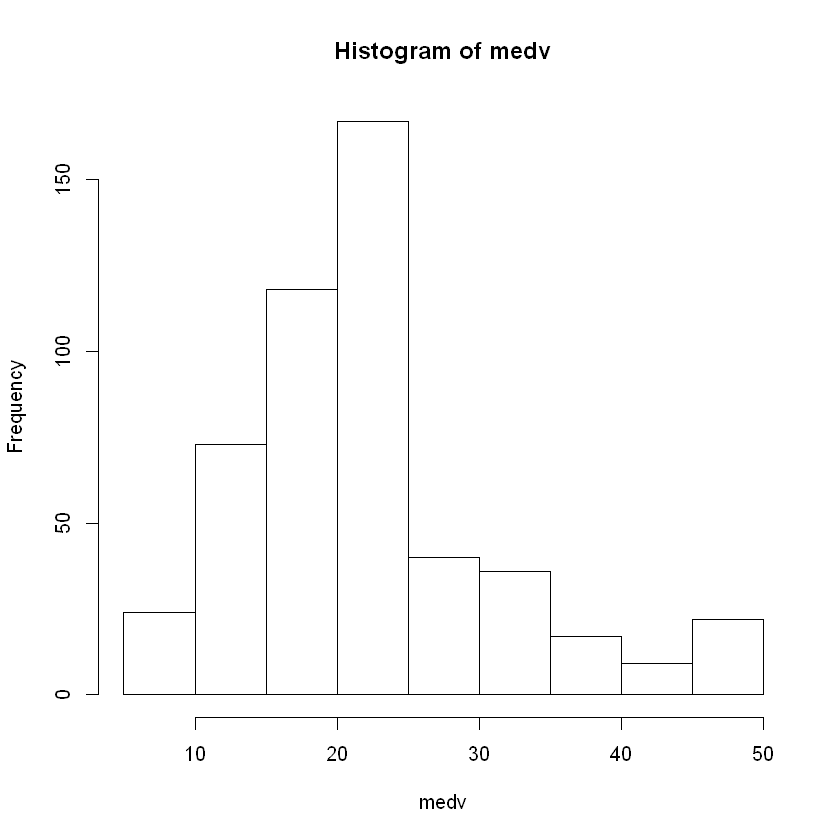In : qqnorm(medv,pch=1,frame=FALSE) qqline(medv,col="blue",lwd=2)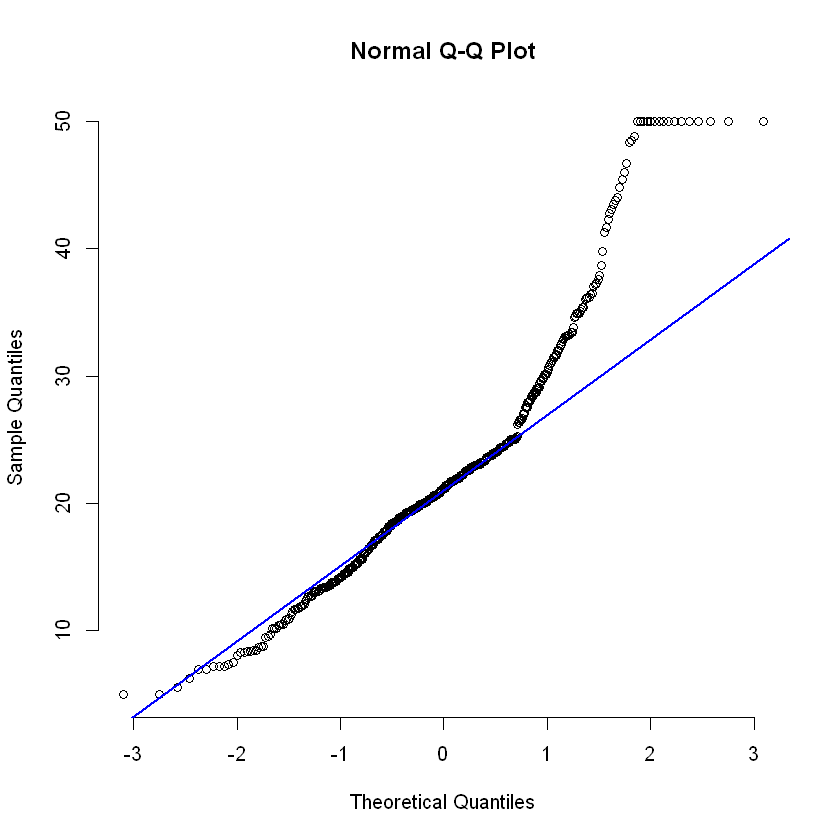In : require(gpairs) gpairs(Boston)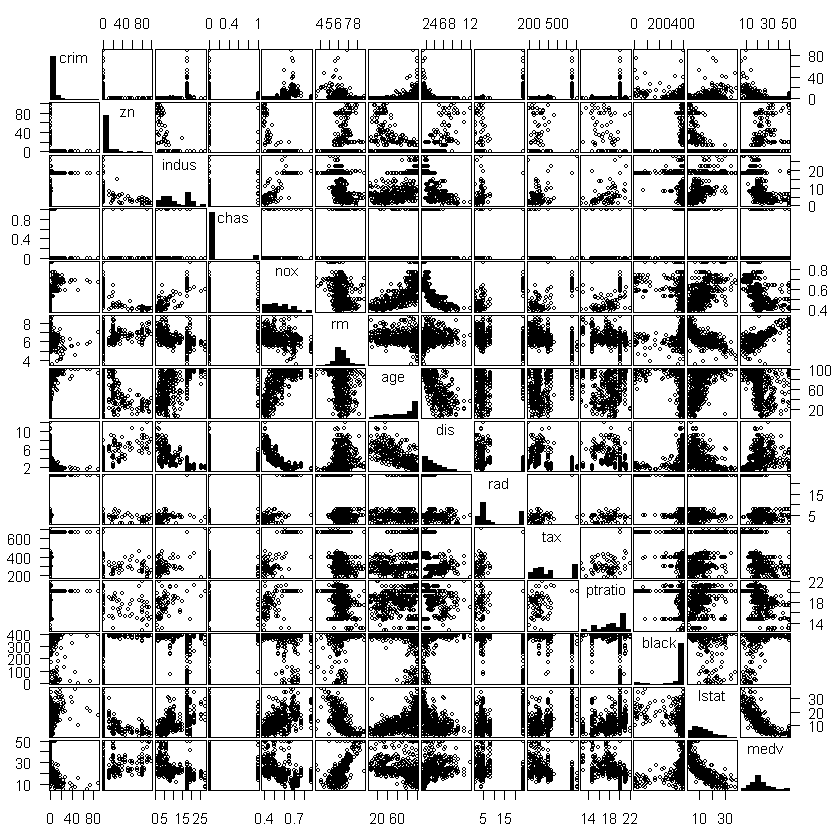Let see how the the med price is distributed based on the variable chas In : plot(zn~medv)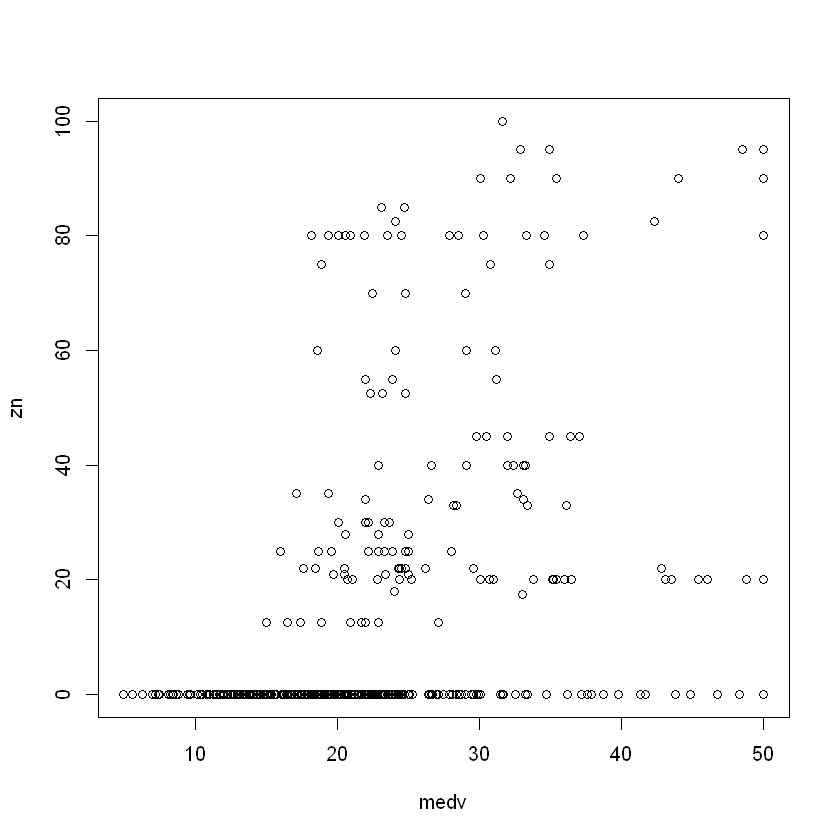In : boxplot(medv~chas)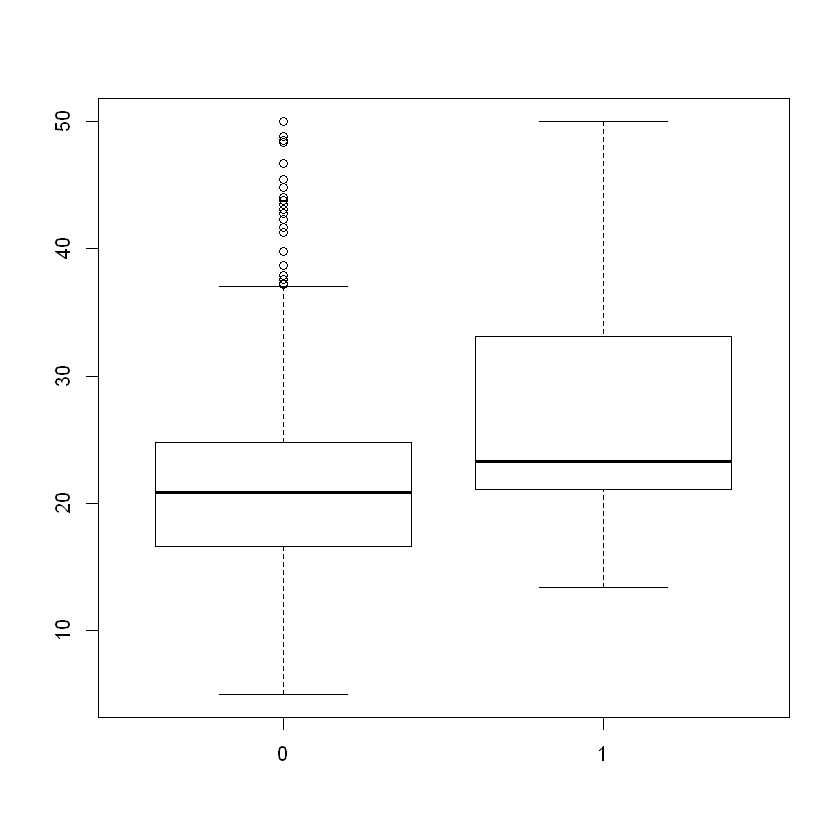In : crim_dummy =ifelse(crim<mean(crim),1,0) table(crim_dummy)  crim_dummy 0 1 128 378  In : boxplot(medv~crim_dummy)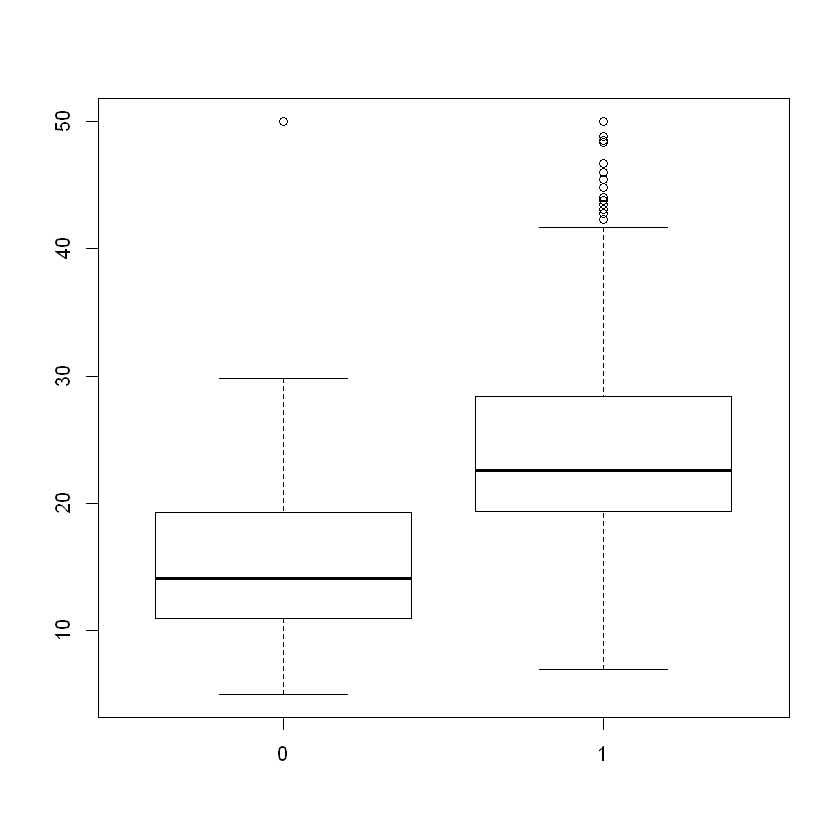The majority of the zn values are zero. That is, many suburbs have no residential land zoned for lots over 25,000 sq ft. In : zn  1. 18 2. 0 3. 0 4. 0 5. 0 6. 0 7. 12.5 8. 12.5 9. 12.5 10. 12.5 11. 12.5 12. 12.5 13. 12.5 14. 0 15. 0 16. 0 17. 0 18. 0 19. 0 20. 0 21. 0 22. 0 23. 0 24. 0 25. 0 26. 0 27. 0 28. 0 29. 0 30. 0 31. 0 32. 0 33. 0 34. 0 35. 0 36. 0 37. 0 38. 0 39. 0 40. 75 41. 75 42. 0 43. 0 44. 0 45. 0 46. 0 47. 0 48. 0 49. 0 50. 0 51. 21 52. 21 53. 21 54. 21 55. 75 56. 90 57. 85 58. 100 59. 25 60. 25 61. 25 62. 25 63. 25 64. 25 65. 17.5 66. 80 67. 80 68. 12.5 69. 12.5 70. 12.5 71. 0 72. 0 73. 0 74. 0 75. 0 76. 0 77. 0 78. 0 79. 0 80. 0 81. 25 82. 25 83. 25 84. 25 85. 0 86. 0 87. 0 88. 0 89. 0 90. 0 91. 0 92. 0 93. 28 94. 28 95. 28 96. 0 97. 0 98. 0 99. 0 100. 0 101. 0 102. 0 103. 0 104. 0 105. 0 106. 0 107. 0 108. 0 109. 0 110. 0 111. 0 112. 0 113. 0 114. 0 115. 0 116. 0 117. 0 118. 0 119. 0 120. 0 121. 0 122. 0 123. 0 124. 0 125. 0 126. 0 127. 0 128. 0 129. 0 130. 0 131. 0 132. 0 133. 0 134. 0 135. 0 136. 0 137. 0 138. 0 139. 0 140. 0 141. 0 142. 0 143. 0 144. 0 145. 0 146. 0 147. 0 148. 0 149. 0 150. 0 151. 0 152. 0 153. 0 154. 0 155. 0 156. 0 157. 0 158. 0 159. 0 160. 0 161. 0 162. 0 163. 0 164. 0 165. 0 166. 0 167. 0 168. 0 169. 0 170. 0 171. 0 172. 0 173. 0 174. 0 175. 0 176. 0 177. 0 178. 0 179. 0 180. 0 181. 0 182. 0 183. 0 184. 0 185. 0 186. 0 187. 0 188. 45 189. 45 190. 45 191. 45 192. 45 193. 45 194. 60 195. 60 196. 80 197. 80 198. 80 199. 80 200. 95 201. 95 202. 82.5 203. 82.5 204. 95 205. 95 206. 0 207. 0 208. 0 209. 0 210. 0 211. 0 212. 0 213. 0 214. 0 215. 0 216. 0 217. 0 218. 0 219. 0 220. 0 221. 0 222. 0 223. 0 224. 0 225. 0 226. 0 227. 0 228. 0 229. 0 230. 0 231. 0 232. 0 233. 0 234. 0 235. 0 236. 0 237. 0 238. 0 239. 30 240. 30 241. 30 242. 30 243. 30 244. 30 245. 22 246. 22 247. 22 248. 22 249. 22 250. 22 251. 22 252. 22 253. 22 254. 22 255. 80 256. 80 257. 90 258. 20 259. 20 260. 20 261. 20 262. 20 263. 20 264. 20 265. 20 266. 20 267. 20 268. 20 269. 20 270. 20 271. 20 272. 20 273. 20 274. 20 275. 40 276. 40 277. 40 278. 40 279. 40 280. 20 281. 20 282. 20 283. 20 284. 90 285. 90 286. 55 287. 80 288. 52.5 289. 52.5 290. 52.5 291. 80 292. 80 293. 80 294. 0 295. 0 296. 0 297. 0 298. 0 299. 70 300. 70 301. 70 302. 34 303. 34 304. 34 305. 33 306. 33 307. 33 308. 33 309. 0 310. 0 311. 0 312. 0 313. 0 314. 0 315. 0 316. 0 317. 0 318. 0 319. 0 320. 0 321. 0 322. 0 323. 0 324. 0 325. 0 326. 0 327. 0 328. 0 329. 0 330. 0 331. 0 332. 35 333. 35 334. 0 335. 0 336. 0 337. 0 338. 0 339. 0 340. 0 341. 0 342. 35 343. 0 344. 55 345. 55 346. 0 347. 0 348. 85 349. 80 350. 40 351. 40 352. 60 353. 60 354. 90 355. 80 356. 80 357. 0 358. 0 359. 0 360. 0 361. 0 362. 0 363. 0 364. 0 365. 0 366. 0 367. 0 368. 0 369. 0 370. 0 371. 0 372. 0 373. 0 374. 0 375. 0 376. 0 377. 0 378. 0 379. 0 380. 0 381. 0 382. 0 383. 0 384. 0 385. 0 386. 0 387. 0 388. 0 389. 0 390. 0 391. 0 392. 0 393. 0 394. 0 395. 0 396. 0 397. 0 398. 0 399. 0 400. 0 401. 0 402. 0 403. 0 404. 0 405. 0 406. 0 407. 0 408. 0 409. 0 410. 0 411. 0 412. 0 413. 0 414. 0 415. 0 416. 0 417. 0 418. 0 419. 0 420. 0 421. 0 422. 0 423. 0 424. 0 425. 0 426. 0 427. 0 428. 0 429. 0 430. 0 431. 0 432. 0 433. 0 434. 0 435. 0 436. 0 437. 0 438. 0 439. 0 440. 0 441. 0 442. 0 443. 0 444. 0 445. 0 446. 0 447. 0 448. 0 449. 0 450. 0 451. 0 452. 0 453. 0 454. 0 455. 0 456. 0 457. 0 458. 0 459. 0 460. 0 461. 0 462. 0 463. 0 464. 0 465. 0 466. 0 467. 0 468. 0 469. 0 470. 0 471. 0 472. 0 473. 0 474. 0 475. 0 476. 0 477. 0 478. 0 479. 0 480. 0 481. 0 482. 0 483. 0 484. 0 485. 0 486. 0 487. 0 488. 0 489. 0 490. 0 491. 0 492. 0 493. 0 494. 0 495. 0 496. 0 497. 0 498. 0 499. 0 500. 0 501. 0 502. 0 503. 0 504. 0 505. 0 506. 0 In : zn.zero<-ifelse(zn==0,1,0) table(zn.zero)  zn.zero 0 1 134 372  In : boxplot(medv~zn.zero)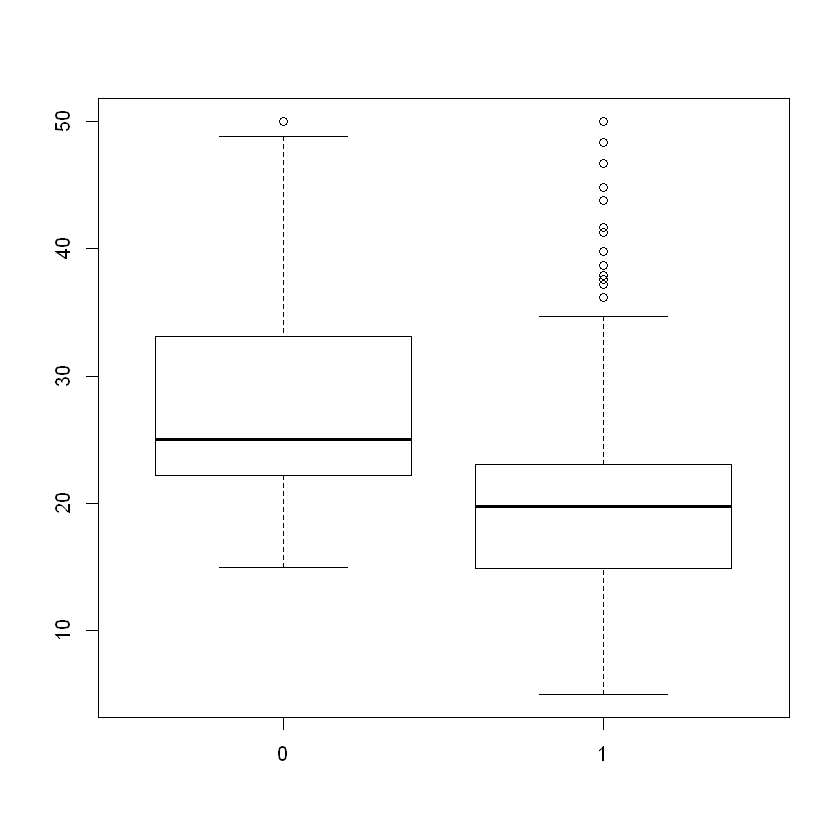In : boxplot(indus[zn.zero==1],indus[zn.zero==0],names=c("Zero Res. Land Zoned", "Some Res. Land Zoned"),main="Proportion of Non-Retail Business Acres")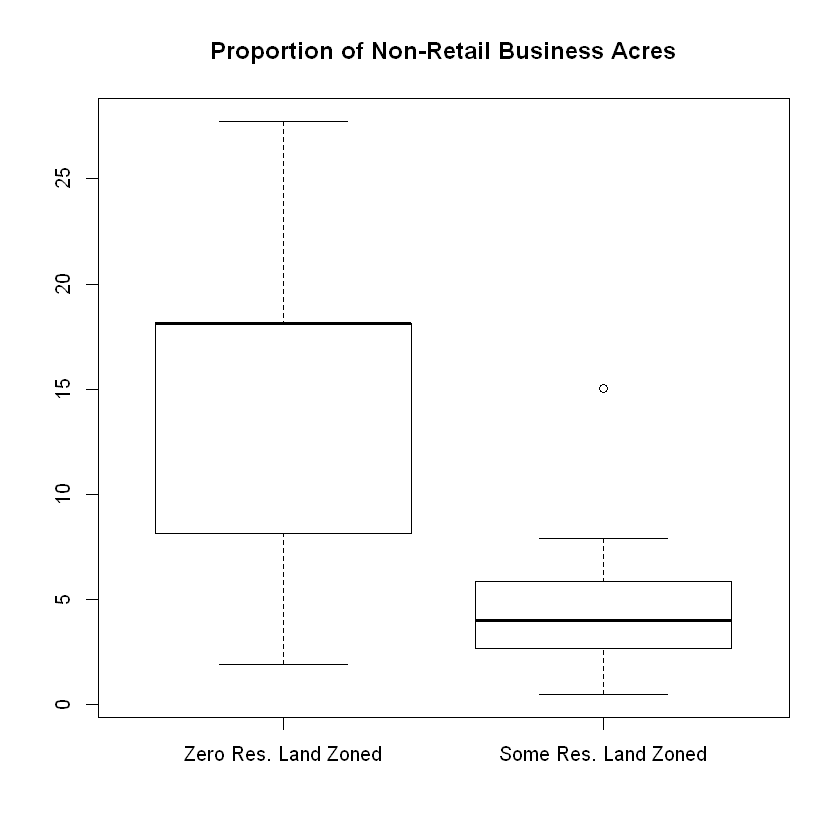The suburbs with no residential land zoned for lots over 25,000 sq ft have a much higher proportion of non-retail business acres. Large lots usually correspond to business parks, strip shopping centers, shopping malls, etc. It would make sense that areas with no zoned lots of this size would have a higher proportion of non-retail businesses. The variance of this group is also much higher, likely due to outliers. The rm variable is real-valued (because it is an average). We create a categorical variable rm2 that rounds the number of rooms to the nearest integer. Let check what are the different values of number of rooms now and how often do each of them occur? In : summary(rm)   Min. 1st Qu. Median Mean 3rd Qu. Max. 3.561 5.886 6.208 6.285 6.623 8.780  In : rm2<-round(rm,0) summary(rm2)   Min. 1st Qu. Median Mean 3rd Qu. Max. 4.000 6.000 6.000 6.267 7.000 9.000  In : sort(unique(rm2))  1. 4 2. 5 3. 6 4. 7 5. 8 6. 9 In : table(rm2)  rm2 4 5 6 7 8 9 5 37 312 125 24 3  Now we use graphs to illustrate the differences (for at least three variables) between towns with a pupil-teacher ratio of less than 20 and towns with a pupil-teacher ratio of greater than or equal to 20. In : summary(ptratio) pt.20<-ifelse(ptratio>=20,1,0) table(pt.20)   Min. 1st Qu. Median Mean 3rd Qu. Max. 12.60 17.40 19.05 18.46 20.20 22.00  pt.20 0 1 305 201  In : m<-matrix(c(1,2,3,4,5,6),ncol=2) layout(m) #Crime Rate: very different distributions; best looked at with histograms hist(crim[pt.20==1],xlab="Per Capita Crime Rate",main="Crime: PT Ratio >= 20") hist(crim[pt.20==0],xlab="Per Capita Crime Rate",main="Crime: PT Ratio < 20") # Proportion of Residential Land zoned: #the huge frequency of zn = 0 makes the distribution hard to graph. Probably more useful to look at the #table of the two indicator variables (zn = 0 yes/no vs. pt.ratio >= 20 yes/no) barplot(table(zn.zero,pt.20),beside=T, names=c("Pt.Ratio >=20", "Pt.Ratio<20"),legend.text=c("None Zoned", "Some Zoned"), main="Residential Zoning vs. PT Ratio") #Proportion of Non-retail business acres per town: very different distributions; best looked at with histograms hist(indus[pt.20==1],xlab="Proportion of Non-Retail Business Acres",main="Indus: Pt Ratio >=20") hist(indus[pt.20==0],xlab="Proportion of Non-Retail Business Acres",main="Indus: Pt Ratio < 20") #Charles River: dummy variable; again we look at the table of the two indicator variables barplot(table(chas,pt.20),beside=T, names=c("Pt.Ratio >=20", "Pt.Ratio<20"),legend.text=c("Bounds River", "Doesn’t Bound River"), main="Charles River vs. PT Ratio")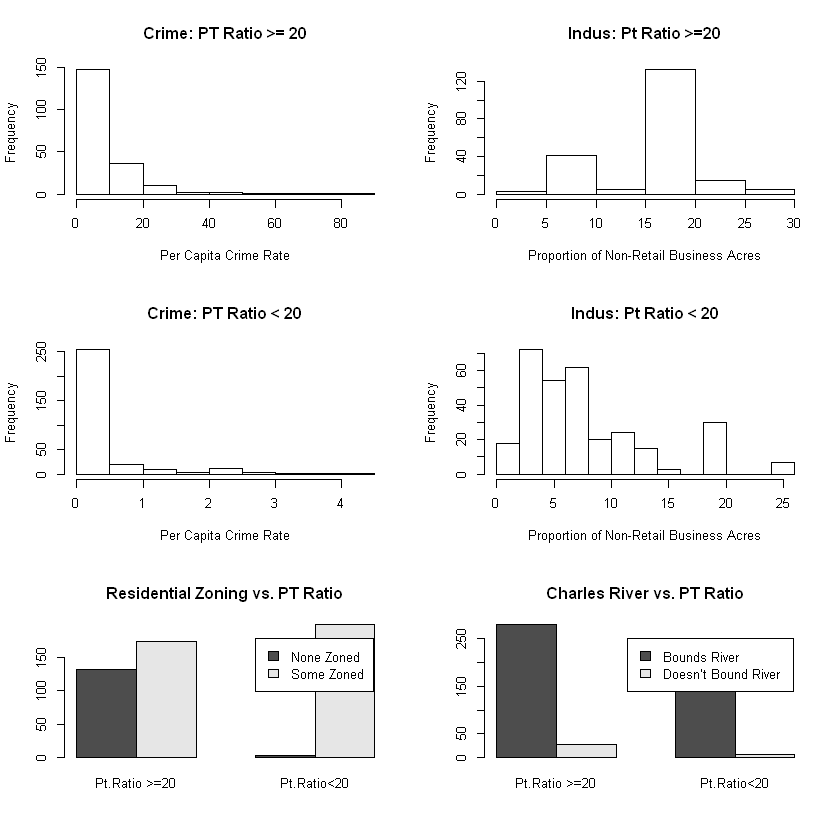The per capita crime rate appears to be much higher in towns with a greater pupil-teacher ratio. The proportion of non-retail business acres is not distributed that much differently when you look at the actual frequencies excepting a very large peak around 15-20% in the suburbs with a large pupil-teacher ratio. Differences in amount of residential land zoned are apparent in suburbs with a low pupil-teacher ratio. The differences between suburbs on the river and off the river do not appear to depend greatly on the pupil-teacher ratio. In : par(mfrow=c(3,2)) #Nitrogen Oxides Concentration (parts per 10 million) boxplot(nox[pt.20==1],nox[pt.20==0],names=c("Pt.Ratio >=20", "Pt.Ratio<20"),main="NOX vs. PT Ratio") #Average number of rooms per dwelling boxplot(rm[pt.20==1],rm[pt.20==0],names=c("Pt.Ratio >=20", "Pt.Ratio<20"),main="Avg # of Rooms vs. PT Ratio") #Proportion of owner-occupied units built prior to 1940 boxplot(age[pt.20==1],age[pt.20==0],names=c("Pt.Ratio >=20", "Pt.Ratio<20"),main="# of Units built pre-1940 vs. PT Ratio") #weighted mean of distances to five Boston employment centers boxplot(dis[pt.20==1],dis[pt.20==0],names=c("Pt.Ratio >=20", "Pt.Ratio<20"),main="Dist to Employment Centers vs. PT Ratio") # Index of Accessibility to radial highways: very different distributions but integer-valued #better to use barplots but we want the ranges to be the same rad1.freq<-rad2.freq<-rep(0,max(rad,na.rm=T)-min(rad,na.rm=T)+1) for(i in 1:506){ if(!is.na(pt.20[i])){ if(pt.20[i]==1) rad1.freq[rad[i]]<-rad1.freq[rad[i]]+1 if(pt.20[i]==0) rad2.freq[rad[i]]<-rad2.freq[rad[i]]+1 } } barplot(rad1.freq,xlab="Index of Accessibility to Radial Highways",names=seq(1,24),col=3,main="Rad: PT Ratio >=20") barplot(rad2.freq,xlab="Index of Accessibility to Radial Highways",names=seq(1,24),col=3,main="Rad: PT Ratio < 20")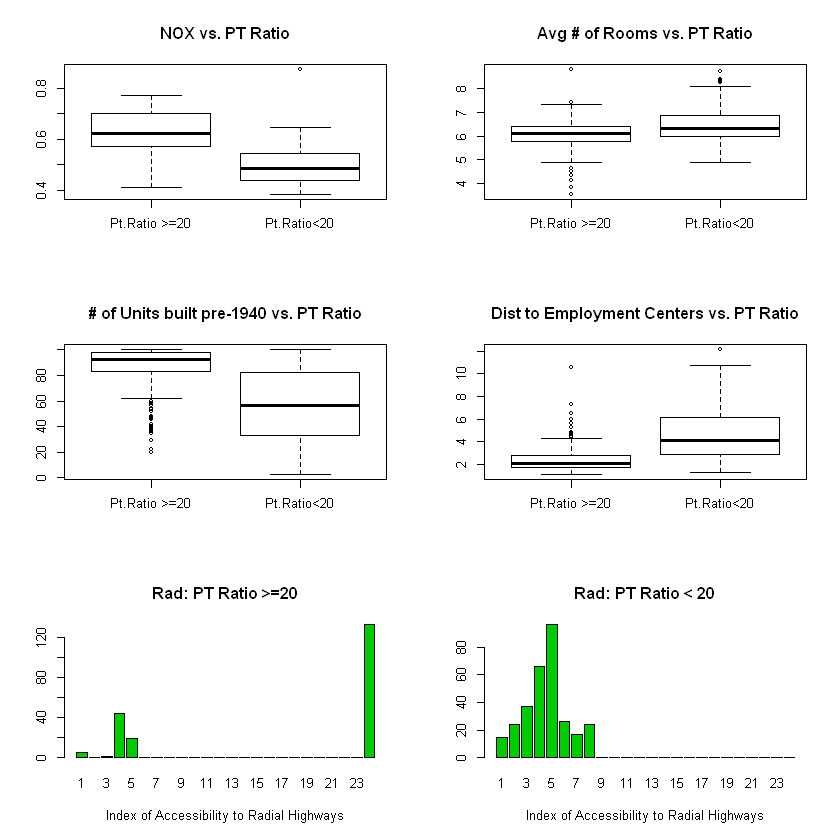In suburbs with a higher pupil-teacher ratio, the nitrogen oxides concentration appears to be higher. The average number of rooms doesn’t seem to be dependent on the pupil-teacher ratio; suburbs with a low pupil-teacher ratio perhaps have slightly larger houses. Suburbs with high pupil-teacher ratios have older houses (but note the large number of outliers) and are closet to employment centers. With regards to accessibility to radial highways, there appears to be a group of high pupil-teacher ratio suburbs with better access. Otherwise, the shape of the distributions is not that different (taking into account the frequencies). The goal is to predict the median value of owner-occupied homes medv based on the given predictors. We start by creating a training set, and fit the tree to the training data In : set.seed (1) train = sample (1: nrow(Boston ), nrow(Boston )/2) tree.boston =tree(medv∼.,Boston ,subset =train) summary (tree.boston )  Regression tree: tree(formula = medv ~ ., data = Boston, subset = train) Variables actually used in tree construction:  "lstat" "rm" "dis" Number of terminal nodes: 8 Residual mean deviance: 12.65 = 3099 / 245 Distribution of residuals: Min. 1st Qu. Median Mean 3rd Qu. Max. -14.10000 -2.04200 -0.05357 0.00000 1.96000 12.60000  Notice that the output of summary() indicates that only three of the variables have been used in constructing the tree. In the context of a regression tree, the deviance is simply the sum of squared errors for the tree. We now plot the tree. In : plot(tree.boston ) text(tree.boston ,pretty =0)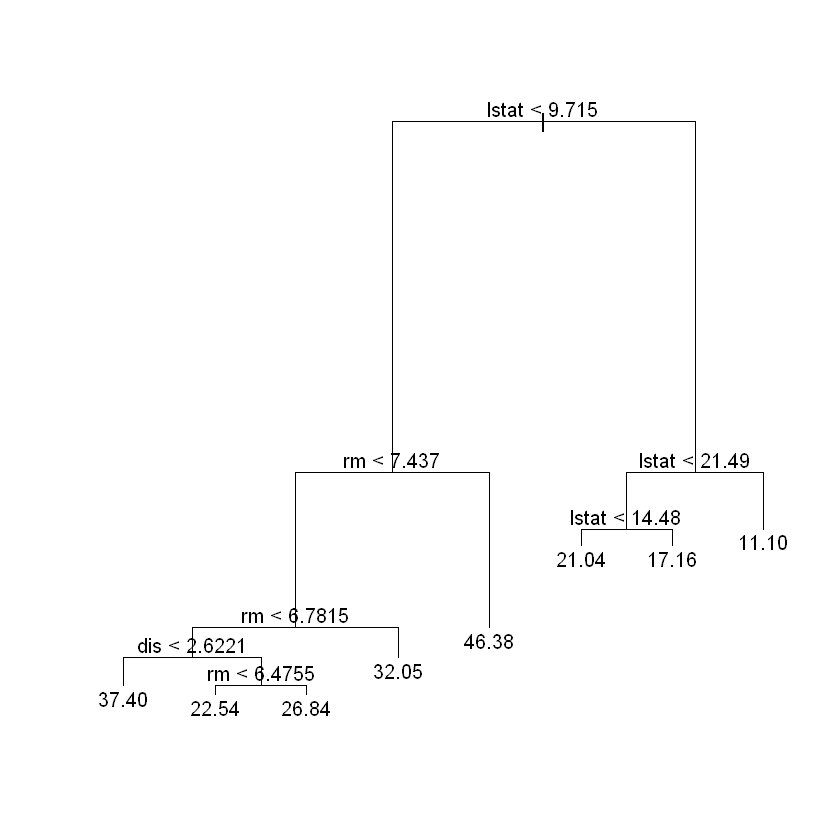The variable lstat measures the percentage of individuals with lower socioeconomic status. The tree indicates that lower values of lstat correspond to more expensive houses. The tree predicts a median house price of$\$46,380$ for larger homes in suburbs in which residents have high socioeconomic status ($rm\geq 7.5$ and $lstat<9.715$).

Now we use the cv.tree()function to see whether pruning the tree will improve performance.

In :
cv.boston =cv.tree(tree.boston )
plot(cv.boston$size ,cv.boston$dev ,type="b")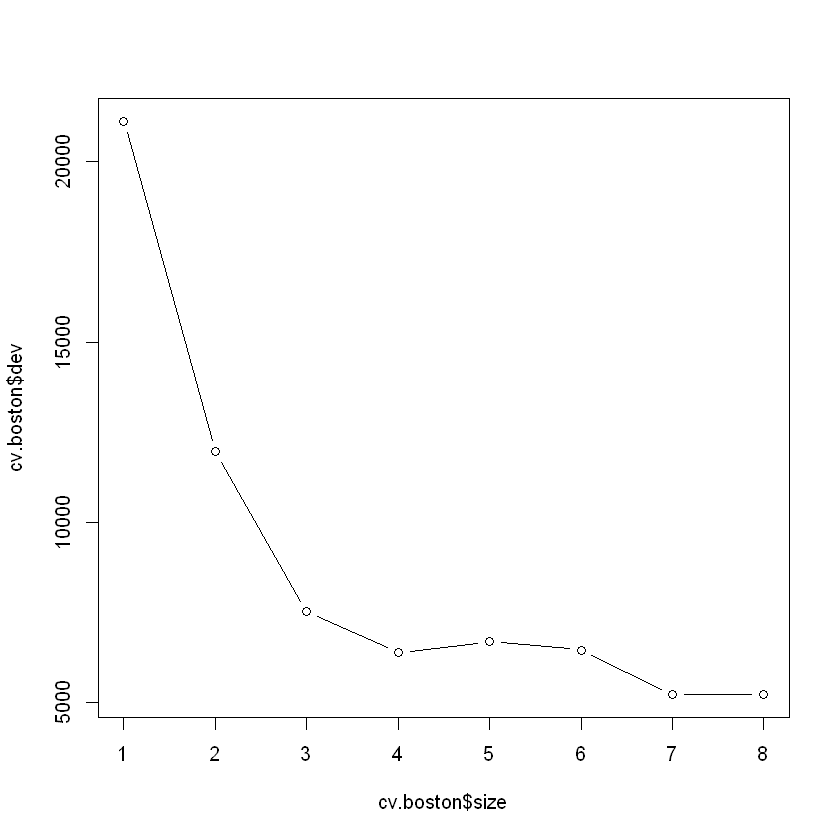In this case, the most complex tree is selected by cross-validation. However, if we wish to prune the tree, we could do so as follows, using the prune.tree() function:

In :
prune.boston =prune.tree(tree.boston ,best =5)
plot(prune.boston )
text(prune.boston ,pretty =0)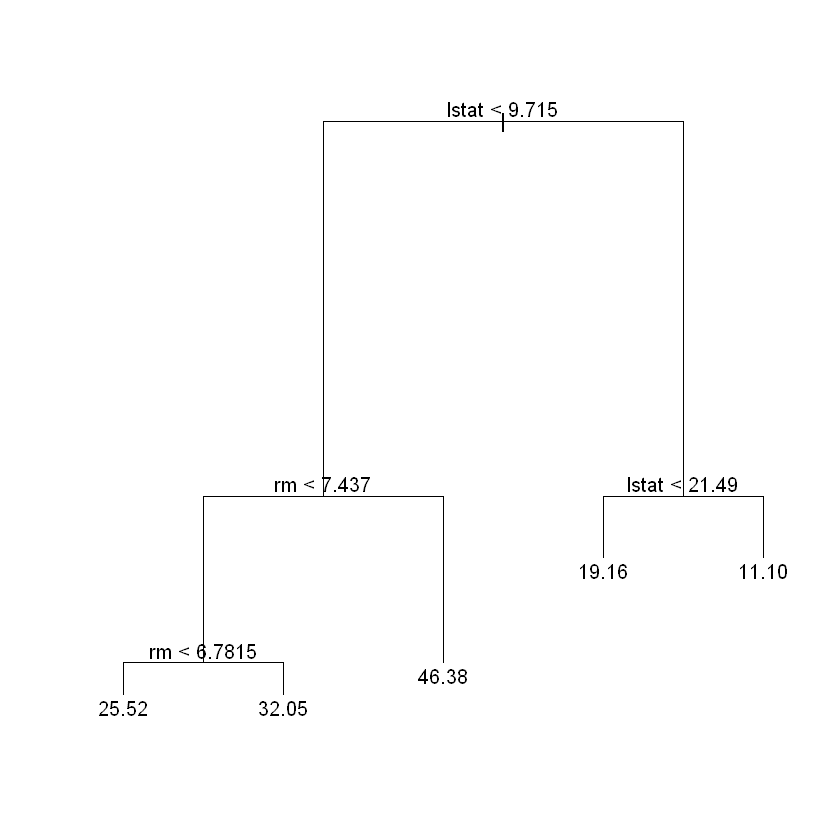In keeping with the cross-validation results, we use the unpruned tree to make predictions on the test set.

In :
yhat=predict(tree.boston ,newdata =Boston [-train ,])
boston.test=Boston [-train ,"medv"]
plot(yhat ,boston.test)
abline (0,1)
ssr.tree<-(yhat -boston.test)^2
round(mean((yhat -boston.test)^2),3)

25.046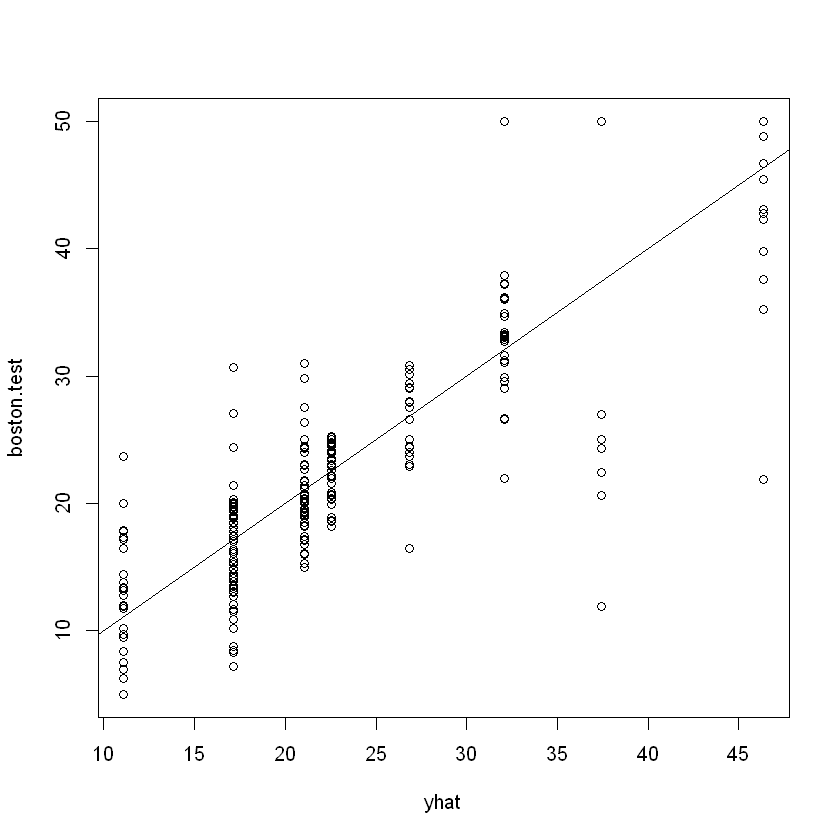In other words, the test set MSE associated with the regression tree is $25.05$. The square root of the MSE is therefore around $5.005$, indicating that this model leads to test predictions that are within around $\$5,005$of the true median home value for the suburb. ## Bagging and Random Forest¶ Here we apply bagging and random forests to the Boston data, using the randomForest package in R. Recall that bagging is simply a special case of a random forest with$m = p$. Therefore, the randomForest() function can be used to perform both random forests and bagging. We perform bagging as follows: In : #install.packages("randomForest") library (randomForest) set.seed (1) bag.boston =randomForest(medv∼.,data=Boston ,subset =train , mtry=13, importance =TRUE) bag.boston  Call: randomForest(formula = medv ~ ., data = Boston, mtry = 13, importance = TRUE, subset = train) Type of random forest: regression Number of trees: 500 No. of variables tried at each split: 13 Mean of squared residuals: 11.02509 % Var explained: 86.65 The argument$mtry=13$indicates that all$13$predictors should be considered for each split of the tree—in other words, that bagging should be done. How well does this bagged model perform on the test set? In : yhat.bag = predict (bag.boston ,newdata =Boston [-train ,]) plot(yhat.bag , boston.test) abline (0,1) ssr.bag<-( yhat.bag -boston.test)^2 mean(( yhat.bag -boston.test)^2)  13.4734864318741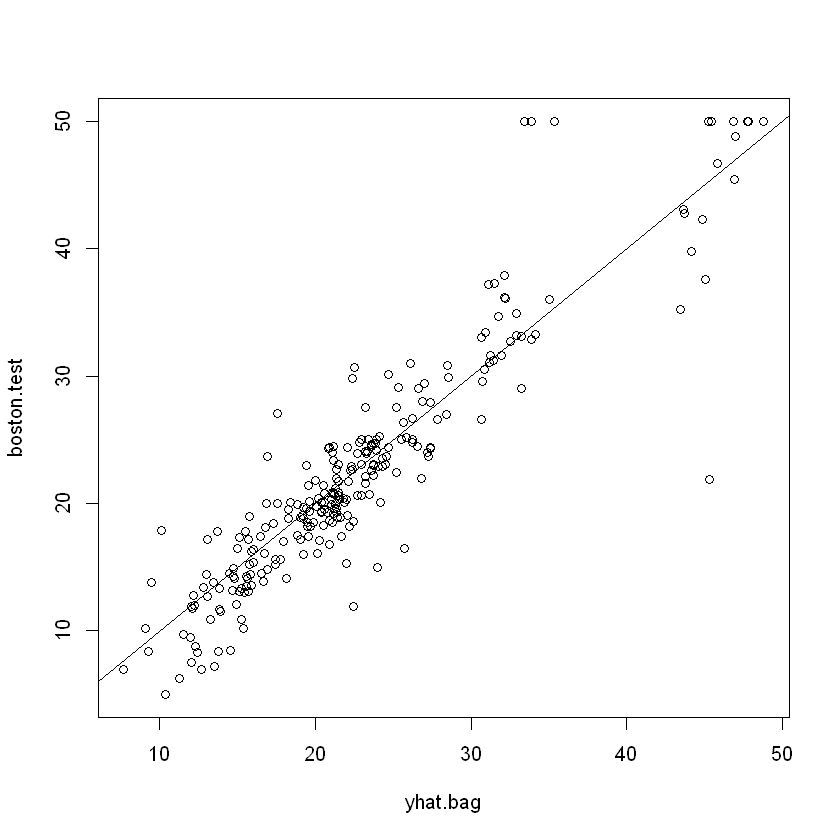The test set MSE associated with the bagged regression tree is$13.16$, almost half that obtained using an optimally-pruned single tree. We could change the number of trees grown by randomForest() using the ntree argument: In : bag.boston =randomForest(medv∼.,data=Boston ,subset =train , mtry=13, ntree =25) yhat.bag = predict (bag.boston ,newdata =Boston [-train ,]) mean(( yhat.bag -boston.test)^2)  13.4306763829776 Growing a random forest proceeds in exactly the same way, except that we use a smaller value of the mtry argument. By default, randomForest() uses$p/3$variables when building a random forest of regression trees. Here we use$mtry = 6$. In : set.seed (1) rf.boston =randomForest(medv∼.,data=Boston ,subset =train , mtry=6, importance =TRUE) yhat.rf = predict (rf.boston ,newdata =Boston [-train ,]) ssr.rf<-( yhat.rf -boston.test)^2 mean(( yhat.rf -boston.test)^2)  11.4802165416298 In : sqrt(11.5)  3.39116499156263 The test set MSE is$11.31\$; this indicates that random forests yielded an improvement over bagging in this case.

Using the importance() function, we can view the importance of each variable.

In :
importance (rf.boston )

%IncMSEIncNodePurity
crim12.547772 1094.65382
zn 1.375489 64.40060
indus 9.304258 1086.09103
chas 2.518766 76.36804
nox12.835614 1008.73703
rm31.646147 6705.02638
age 9.970243 575.13702
dis12.774430 1351.01978
tax 7.624043 453.19472
ptratio12.008194 919.06760
black 7.376024 358.96935
lstat27.666896 6927.98475

Two measures of variable importance are reported. The former is based upon the mean decrease of accuracy in predictions on the out of bag samples when a given variable is excluded from the model. The latter is a measure of the total decrease in node impurity that results from splits over that variable, averaged over all trees. In the case of regression trees, the node impurity is measured by the training RSS. Plots of these importance measures can be produced using the varImpPlot() function.

In :
varImpPlot (rf.boston )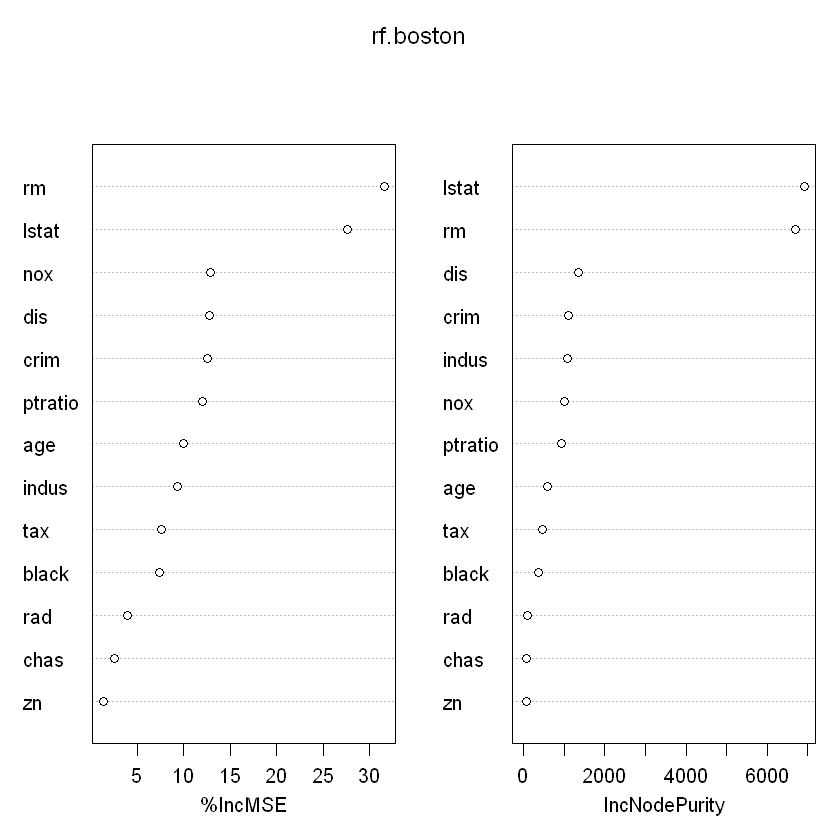The results indicate that across all of the trees considered in the random forest, the wealth level of the community (lstat) and the house size (rm) are by far the two most important variables.

Now let run linear model and compare tree based models with the linear model

In :
lm.boston<-lm(formula = medv ~ crim + chas + nox + rm + dis + rad + tax +ptratio + black + lstat, data = Boston)

In :
yhat.lm = predict (lm.boston ,newdata =Boston [-train ,])

In :
ssr.lm<-( yhat.lm -boston.test)^2

In :
mean(ssr.lm)

24.6497637242437
In :
ssr.tree= rnorm(100,15,8)
ssr.bag= rnorm(100,13,5)
ssr.rf= rnorm(100,10,4)
ssr.lm= rnorm(100,15,13)

In :
boxplot(ssr.tree,ssr.bag,ssr.rf,ssr.lm,names=c("Tree","Bag","RF","LM"),ylim=c(0,100),col=c("blue","green","green","purple"))